ModeratorModeratorAnswer: “RADAR” is an acronym for radio detection and ranging, which is an object-detection system that uses  radio waves to determine the range, angle, or velocity of objects. A radar system consists of a transmitter producing electromagnetic waves in the radio or microwaves domain, an emitting antenna, a receiving antenna (separate or the same as the emitting antenna) to capture any returns from objects in the path of the emitted signal, a receiver, and processor to determine properties of the object.

Question 2: What are the different types of radar?

Answer: Radars are classified according to their functionality as shown in Figure 1.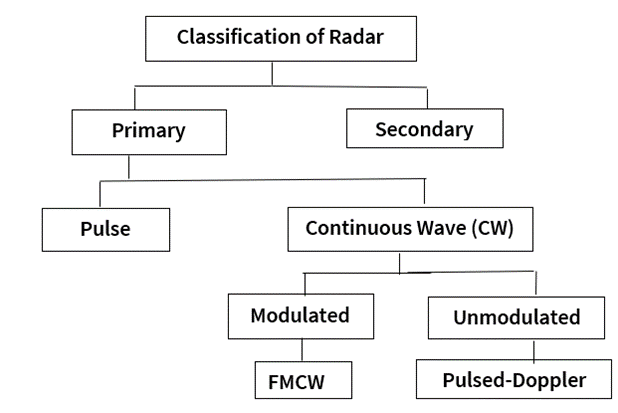Primary and secondary radar systems: A primary radar transmits an electromagnetic signal and receives a reflection of the same signal that it transmits. In a secondary radar, when the transmitted signal strikes the target and the target responds with a coded signal using a transponder.

Pulse and continuous wave (CW) radar systems: A pulse radar transmits a high-power signal, waits for the reflected signal to be collected, and then transmits another signal. However, a CW radar transmits a known stable frequency continuous wave at all times.

Modulated radar: A modulated radar, which is also called as a frequency modulated continuous wave (FMCW) radar, is a system that measures the range of the target using a technique known as frequency modulation. In frequency modulation, the frequency of the electromagnetic wave is increased linearly with time. This kind of signal whose frequency increases linearly with time is called a chirp. The FMCW system measures the instantaneous difference between the transmitted and reflected signal frequencies Δf, which is directly proportional to the time difference Δt of the reflected chirp. The time difference is used to calculate the range of the target. Infineon’s XENSIV™ BGT60TR13C MMIC is an example of a 60 GHz FMCW radar.

Unmodulated radar: An unmodulated continuous wave radar measures the instantaneous rate of change of the target's range by calculating the Doppler shift of the reflected signal. The Doppler shift is a phenomenon, where the frequency of the electromagnetic wave changes because of the movement of either the transmitter, the target, or both. A Pulsed-Doppler radar has the same functionality as an always enabled Doppler radar but is only active for short pulse periods (acting like a pulse radar) while the radar data is sampled to save power. Infineon’s DEMO SENSE2GOL PULSE and DEMO BGT60LTR11AIP are exemplary pulsed-Doppler radar systems.

Question 3: What are the main components of a radar system?

Answer: A typical radar system consists of a transmitter, receiver, RF generator, amplifiers, mixer, and analog-to-digital converters (ADC) as shown in Figure 2.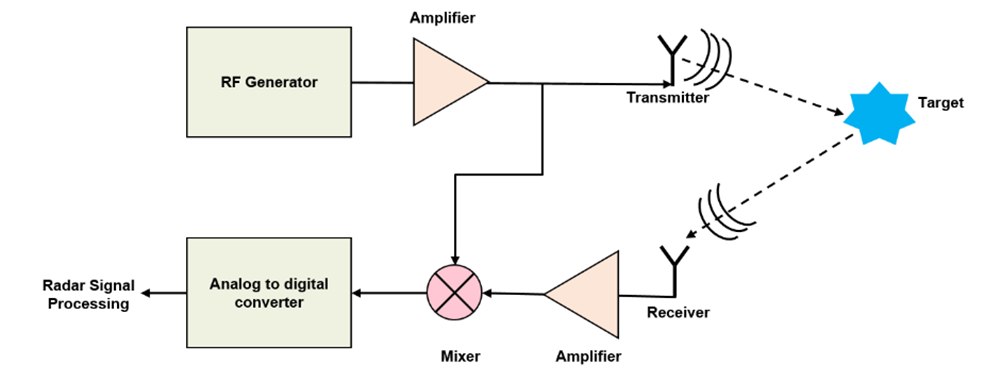Figure 2 Radar system block diagram

An electromagnetic wave generated by the RF generator is amplified and then transmitted through the transmitter antenna. The wave travels through air at a constant speed, approximately at the speed of light and gets reflected once it strikes a target. The reflected signal is collected by the receiver, amplified, and fed to a mixer where it gets mixed with the transmitted signal. The resulting signal from the mixer is called as intermediate frequency (IF) signal, whose instantaneous frequency is equal to the difference of the instantaneous frequencies of the two input signals and phase is equal to the phase difference of the two input signals. The IF signal is digitized through an ADC, which can be further processed by a computer.

Question 4: What is the Doppler effect?

Answer: The Doppler effect comes into play when wave fronts, transmitted by a wave generator (sound, microwaves, light and so on.) hit a moving target. Depending on the direction of the motion of this object, the wave fronts are either compressed or diluted, which finally means a shift in frequency. The signal, shifted in frequency and reflected, is subtracted from the unchanged transmit signal in a relatively simple mixer (otherwise called homodyne mixing) and results in a sinusoidal intermediate frequency (IF). Note that it does not matter whether the sensor moves relatively to the object, or the object moves relatively to the sensor. The Doppler effect is illustrated in Figure 3.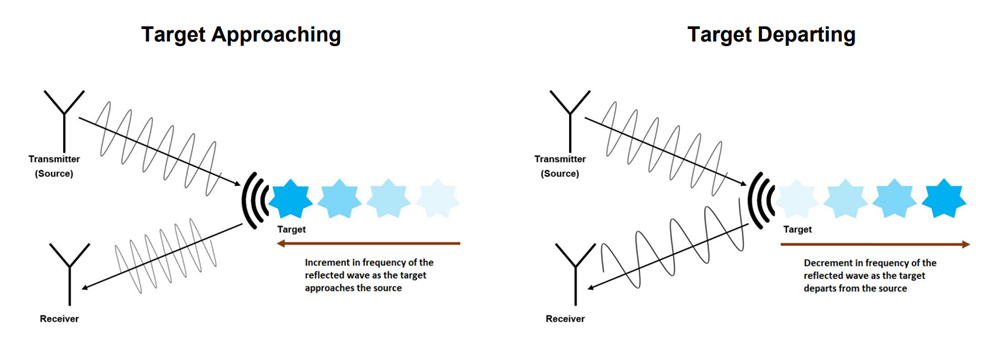Figure 3 Doppler effect

The changes in frequencies in Figure 3 are a result of the Doppler effect and the frequency shift is known as the Doppler frequency.

Question 5: What are the frequencies that can be employed in radars?

Answer: Radar systems have been operated at frequencies as low as 3 MHz and as high as 300 GHz; laser radars operate at frequencies on the order of 1012 to 1015 Hz, corresponding to wavelengths on the order of 0.3 to 30 μm. However, most radars operate in the microwave frequency region of about 200 MHz to about 95 GHz, with corresponding wavelengths of 0.67 m to 3.16 mm. From the whole spectrum for radar frequencies from 3 MHz to 300 GHz, specific frequencies are allocated by international agreement for specific radar operations. The application-based classification of radar frequencies is as shown in Table 1.

Table 1 Application-based classification of radar frequencies

 Application Frequency Wavelength Diverse 200 GHz 150 µm Automotive 77 GHz 3.9 mm Consumer/Industrial 60 GHz 5 mm Consumer/Industrial 24 GHz 1.25 cm UWB 9 GHz 3.3 cm LTE Advanced 6 GHz 5 cm Microwave Oven 2.4 GHz 12.5 cm

Infineon’s millimeter wave (mmWave) radar portfolio is designed to support a wide range of industrial, consumer, and automotive applications, offering both FMCW as well as Doppler radar sensors supporting 24 GHz, 60 GHz, and 77/79 GHz.

Question 6: What are some of the applications of Infineon radars?

Answer: Infineon’s radar sensors serve a wide range of automotive and IoT applications. Most commonly, radar sensors are used in motion detection triggering systems such as indoor and outdoor lighting solutions, automatic doors, camera and security systems, or smart home devices. Additionally, object detection can be ensured through the application of radar sensor and motion sensor ICs, since they can determine the direction of a moving object, speed of an object, distance, and depending on the antenna configuration, even the position of a moving object.Question 7: What are the various radar processing technologies and their capabilities?

Answer: There are three main types of radar processing technologies (otherwise known as, radar operating modes), which are generally employed: Doppler, frequency shift keying (FSK) and frequency modulated continous wave (FMCW). They differ mainly on the type of signal that is transmitted for impinging on the target as shown in Figure 5.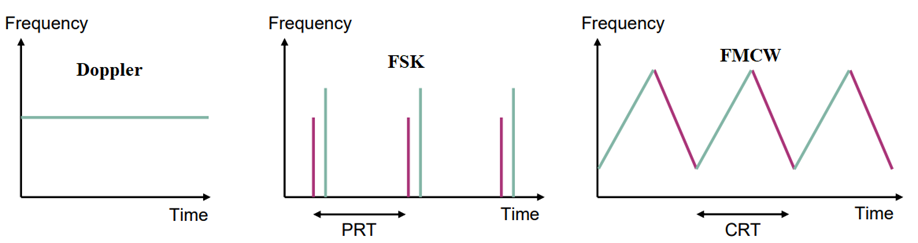A radar operating in Doppler mode will transmit a constant frequency signal for getting the target information, whereas the radar which operates in FSK mode will send out pulses and as for FMCW mode, the transmitted signal will be in the form of chirps (rising frequency slopes are known as up-chirp and falling frequency slopes are known as down-chirp; the signal could be any one type of chirp or a combination of both). Additionally, pulse repetition time (PRT) indicates the duration in FSK after which the pulse sequence is repeated. Similarly, in FMCW mode, the chirp repetition time (CRT) indicates the duration after which a chirp sequence is repeated.

The different radar processing technologies and their capabilities are shown in Figure 6.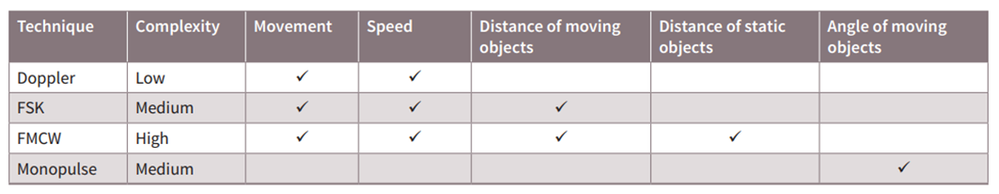Figure 6 Radar processing technologies and capabilities

Question 8: What is the fundamental equation behind the radar operation?

Answer: In a radar system, the EM waves are transmitted from the transmit antenna and propagate in space until they hit a target. The transmitted EM waves are then reflected by the target and are received by the receive antenna. To detect this reflected signal, it must be higher than the smallest detectable signal of a radar system. This minimum signal is usually compared to the noise level and this ratio is referred to by the minimum signal-to-noise ratio (SNRmin). The amount of signal power received is quite critical to the radar’s operation as it defines the difference between detectable and undetectable targets. This value is governed by the fundamental radar equation, which is given as: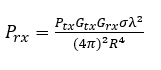where,

Prx is the power of the received signal after the transmitted signal impinges on the target

Ptx is the power of the transmitted signal

Gtx and Grx are the gains of transmitter and receiver antennas respectively

R is the distance between the radar and the target

λ is the wavelength of the transmitted signal

σ is the cross-sectional area of the target

The radar equation shows how the received power is governed by multiple variables. As expected, the power drops very rapidly with distance, which is governed by the term  .
The power is also governed by Gtx and Grx, which are a measure of efficiency of the antennas and how much the antennas focus the energy on the direction of the target.

Also, diverse targets reflect EM waves differently. A big metal object reflects much more energy than a small tennis ball. This is accounted for in the equation with the radar cross section term σ. Additionally, λshows the dependency of the received power on the wavelength and frequency. Considering the equation, this means the higher the frequency the lower the power received.

Question 9: What is range resolution and how is it determined?

Answer: Range resolution is the ability of a radar system to distinguish between two or more targets on the same bearing but at different ranges. The modulation bandwidth (BW) used in the system determines the range resolution. For 24 GHz with 250 MHz BW, the range resolution is c /(2*BW) = 3x108 / (2*250 MHz) = 60 cm.

Question 10: How is range resolution different from range accuracy?

Answer: Accuracy or range measurement accuracy is determined by how well the system is designed. Accuracy is when you are measuring an object and if the object moves a short distance, for example 10 cm, the radar can measure this change with some tolerance. It is characterized by the root-mean square value (RMS) measurement, which is mostly dominated by signal-to-noise ratio (SNR) dependent error. Accuracy can be improved by optimizing SNR and increasing the sampling resolution and rate.

Question 11:  What are the I and Q signals obtained from radars?

Answer: IQ signals in the realm of radar and electrical engineering are signals that have the same amplitude and frequency but are shifted 90° or a quarter cycle relative to each other.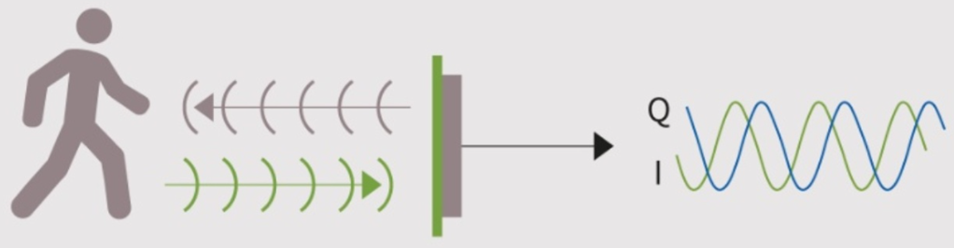Figure 7 IQ signal

Radar systems will have either real receive stages or complex ones. A real stage would only have one component of the received signal while a complex stage will generate both the I and Q components. There are several advantages of generating the IQ signals and using them for further analysis, however, in the case of Doppler radar, the IQ signals serve a more basic purpose.

Since a real mixer used in a Doppler radar receiver will give the absolute frequency shift without a direction or sign, it becomes impossible to determine the direction of travel of the target. Using an IQ mixer, which generates both the I and Q signals, and by checking which of the signals is leading in phase, the direction of travel can be determined.

Question 12: What are the factors that determine whether a target at a particular distance can be identified by a radar or not?

Answer: Identification of a target at a particular distance by the radar primarily depends on the radar cross-section (RCS) of the target, how well this target reflects the radar energy back based on the transmitted energy, and the amount of transmitted energy from the system (EIRP).

Question 13: What kind of angular coverage can be obtained by radar?

Answer: The angular coverage that can be obtained by radar is dependent on the antenna and how it is designed to radiate outward from the sensor. The antenna has a certain angular coverage that can be obtained by its radiation pattern or polar plot. From these data, you can check the efficiency of the antenna along with its gain and half-power beam width.

Question 14: Infineon provides 24 GHz and 60 GHz sensors for industrial, consumer, and IoT based applications. What factors decide which sensor should be used for a particular application?

Answer: In the radar 24 GHz range, the bandwidth for FMCW radar operations covers 250 MHz within the regulated ISM band. In the radar 60 GHz range, an unlicensed ultra-wideband of up to 7 GHz can be used for short-range applications. Consequently, 60 GHz FMCW IoT radar systems can offer a better resolution and therefore allow additional use cases such as human tracking and segmentation. Even gesture sensing, material classification, or the monitoring of various vital functions (respiration, heartbeat, or even blood pressure) is possible with IoT radar technology due to micro-motion detection. Some of the parameters that can be considered while selecting a suitable radar for an application are shown in Figure 8.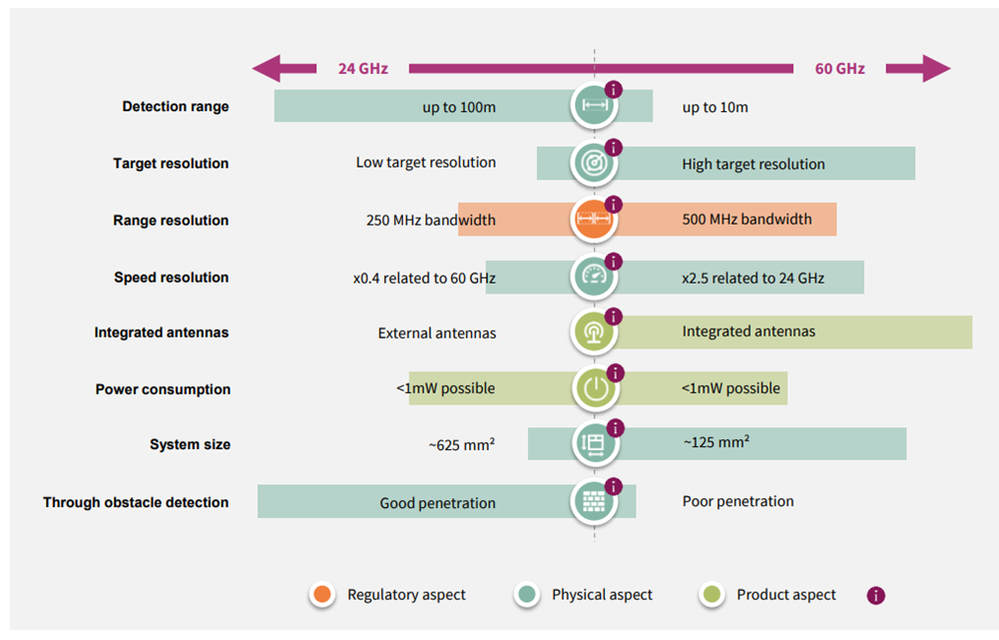Figure 8 Features of 24 GHz vs 60 GHz radar sensors

Question 15:
What are some of the factors that influence the maximum detection range of radars?

Answer: The frequency, peak power, pulse length, and beam width are some of the deciding factors for maximum detection range in radars. The higher the frequency of the radar, the higher also the attenuation. In effect, lower radar frequencies are much more capable of providing higher detection ranges. The peak power of a radar is its useful power. Range capabilities of the radar increase with peak power. The pulse length also has direct influence in the range capability of radar as greater is the pulse length, more will be the transmitted energy. As for beam width, the detection range is greater if the beam is more concentrated.

Question 16: How does radar measure range, speed, and angle of the target?

Answer: By measuring time delay ( ), Doppler frequency shift ( ) of the transmitted signal, and phase difference ( ) between multiple receiving channels that has enough signal-to-noise ratio (SNR), we can estimate the range, Doppler speed, and the angle of the target as shown in Figure 9.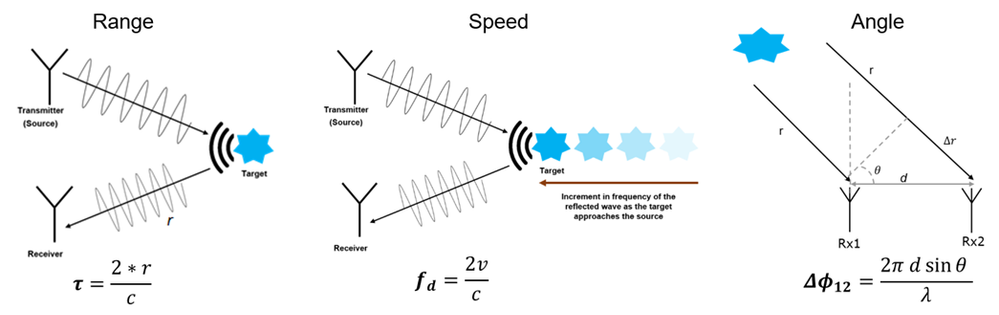Figure 9 Measuring range, speed, and angle in radars

Question 17:

Answer: The radar provides unique advantages over alternative technologies as shown in Figure 10, which compares the radar sensors with the infrared, ultrasonic, and laser sensors.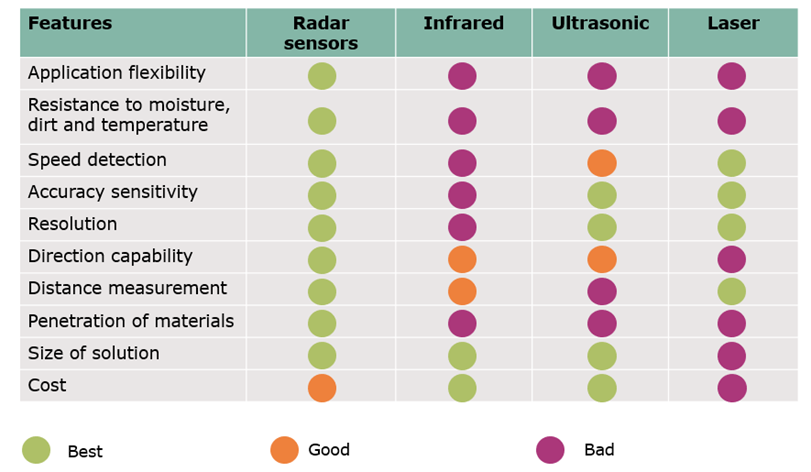Figure 10 Technology Comparison

Question 18: What is the significance of threshold in radars?

Answer: The detection threshold is an important parameter in radar, which acts as the main deciding factor in classifying whether a target is present or not. The classification of received radar signals based on the detection threshold is as shown in Figure 11.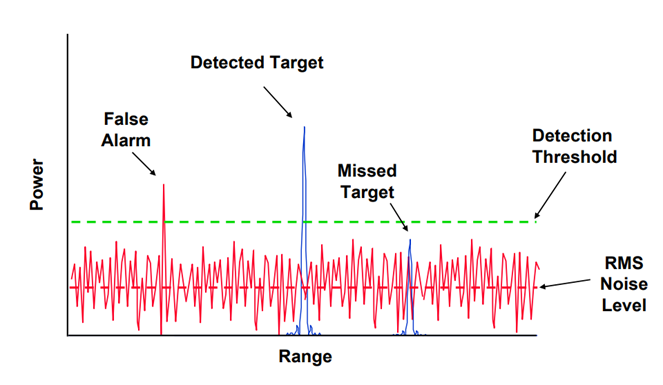Figure 11 Concept of detection threshold in radars

References:

59 Views